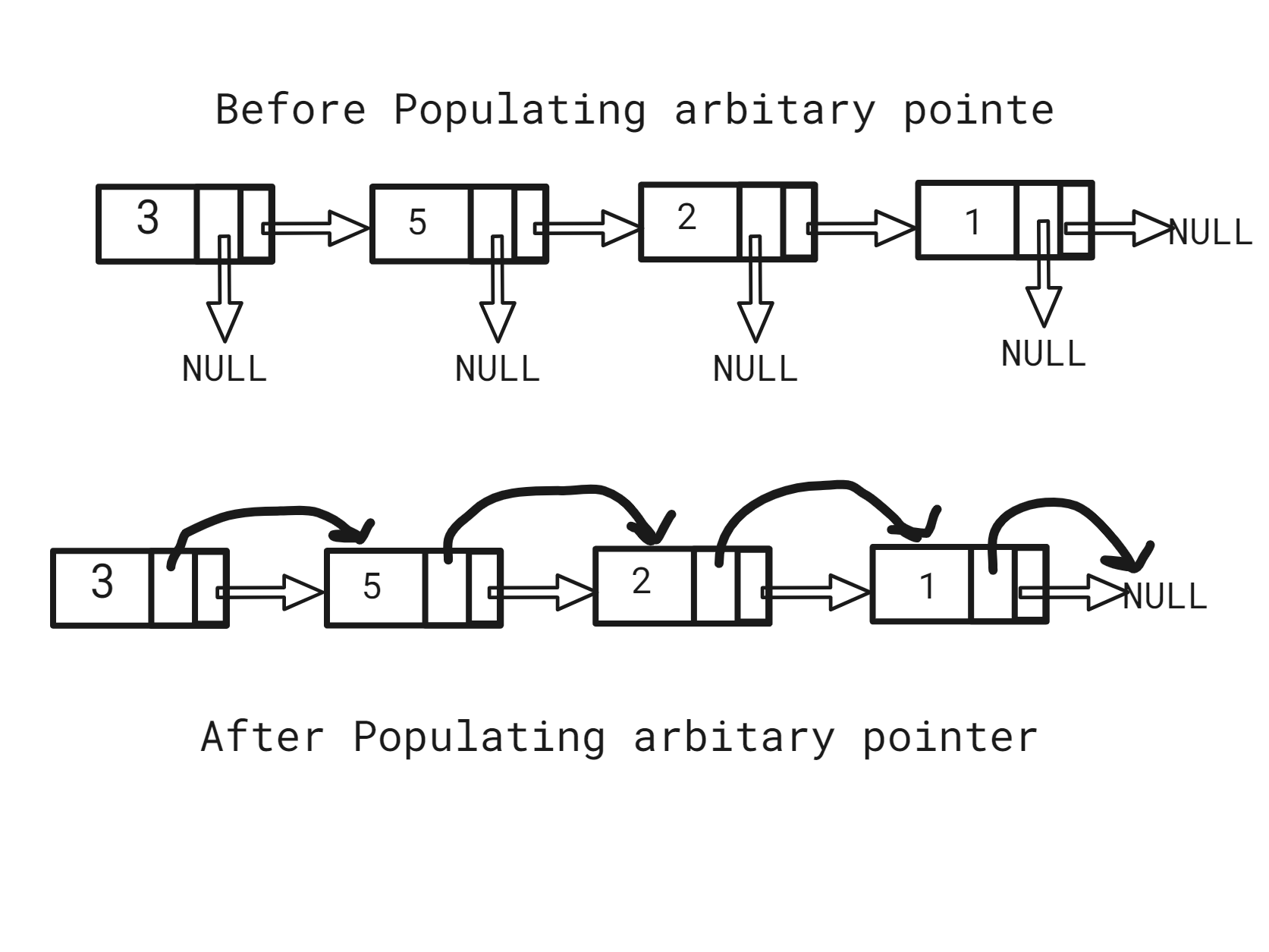Point to Greatest Value Node

Posted: 1 Dec, 2020
Difficulty: Easy

PROBLEM STATEMENT

More formally, each Linked List node has three attributes ‘data’, which is the value of the node, the ‘next’ pointer, which points to the next node, and an ‘arbit’ pointer which is initially NULL.

Note:
You need to return the head after populating the ‘arbit’ pointer.

For example:

Given ‘head’ as 3 -> 5 -> 2 -> 1.After populating the arbitrary pointer, each node's ‘arbit’ pointer would point to 5 2 1 -1 respectively.
Input format:
The first line of input contains an integer ‘T’ denoting the number of test cases.

The second line of each test case contains space-separated integers, denoting the elements in linked list nodes, and -1 denotes the end of the linked list.
Output Format :
For each test case, print integers denoting the value of the nodes being pointed by the arbitrary pointer of that node, if the arbitrary pointer points to NULL, print -1.
Note:
You are supposed to return the head of the linked list, whose arbitrary pointer is populated.

You are not required to print the expected output; it has already been taken care of. Just implement the function.
Constraints:
1 <= ‘T’ <= 10
1 <= ‘N’ <= 5000
0 <= ‘data’ <= 10 ^ 4 and ‘data’ != -1

Time Limit: 1sec.Approach 1

The idea is to loop for every node and find the node with the greatest value with respect to the current node.

The steps are as follows:

• Maintain a ‘tempNode’ which points at the 'head'.
• While ‘tempNode’ is not NULL:
• Maintain a ‘currentNode’ which points to the next of ‘tempNode’, a variable ‘mxVal’ which helps to find the max value towards the right of ‘currentNode’, and ‘maxValNode’, which points to the node with maximum value.
• Point the ‘arbit’ pointer of ‘tempNode’ to ‘maxValNode’.